Courses

# Projectile Motion JEE Main(Part - 3) - Physics, Solution by DC Pandey NEET Notes | EduRev

## DC Pandey (Questions & Solutions) of Physics: NEET

Created by: Ciel Knowledge

## NEET : Projectile Motion JEE Main(Part - 3) - Physics, Solution by DC Pandey NEET Notes | EduRev

The document Projectile Motion JEE Main(Part - 3) - Physics, Solution by DC Pandey NEET Notes | EduRev is a part of the NEET Course DC Pandey (Questions & Solutions) of Physics: NEET.
All you need of NEET at this link: NEET

Objective Questions (Level 1)
Single Correct Option

Ques 1: A particle has initial velocity,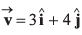and a constant force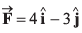acts on it. The path of the particle is
(a) straight line
(b) parabolic
(c) circular
(d) elliptical
Ans: circular
Sol: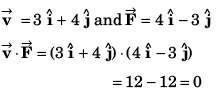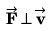Path of the particle is circular.
Option (c) is correct.

Ques 2: Identify the correct statement related to the projectile motion.
(a) It is uniformly accelerated everywhere
(b) It is uniformly accelerated everywhere except at the highest position where it is moving with constant velocity
(c) Acceleration is never perpendicular to velocity
(d) None of the above
Ans: It is uniformly accelerated everywhere
Sol: Projectile motion is uniformly accelerated everywhere even at the highest point.
Option (a) correct.
Option (b) incorrect.
At the highest point acceleration is perpendicular to velocity.
Option (c) incorrect.

Ques 3: A ball is projected with a velocity 20 ms -1 at an angle to the horizontal. In order to have the maximum range. Its velocity at the highest position must be
(a) 10 ms-1
(b) 14 ms-1
(c) 18 ms-1
(d) 16 ms-1
Ans: 14 ms-1
Sol: For range to be maximum
θ = 45°
i.e.,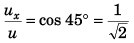or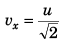(∴ vx = ux)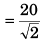= 14.14 m/s = 14 m/s (approx)
Option (b) is correct.

Ques 4: Two bodies are thrown with the same initial velocity at angles θ and (90° - 0) respectively with the horizontal, then their maximum heights are in the ratio
(a) 1 : 1
(b) sin θ : cos θ
(c) sin2 θ : cosθ
(d) cos θ : sin θ
Ans: sin2 θ : cosθ
Sol: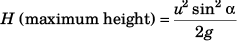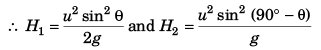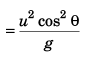Thus,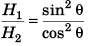Option (c) is correct.

Ques 5: A gun is firing bullets with velocity v0 by rotating through 360° in the horizontal plane. The maximum area covered by the bullets is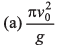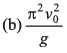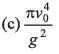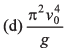Ans: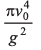Sol: Equation to trajectory is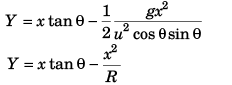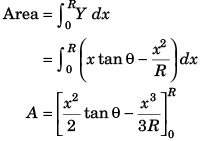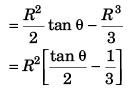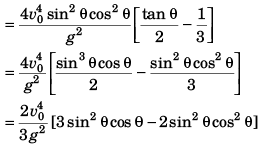Ques 6: A body is projected at an angle 60° with the horizontal with kinetic energy K. When the velocity makes an angle 30° with the horizontal, the kinetic energy of the body will be
(a) K/2
(b) K/3
(c) 2K/3
(d) 3K/4
Ans: K/3
Sol: v cos φ = u cos θ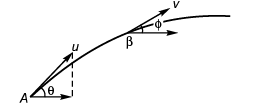or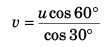or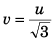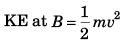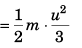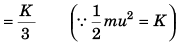∴ Option (b) is correct.

Ques 7: The range of a projectile at an angle 0 is equal to half of the maximum range if thrown at the same speed. The angle of projection 0 is given by
(a) 15°
(b) 30°
(c) 60°
(d) data insufficient
Ans: 15°
Sol: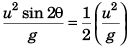or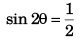or 2θ = 30°
∴ θ = 15°
Option (a) is correct.

Ques 8: If T1 and T2 are the times of flight for two complementary angles, then the range of projectile R is given by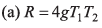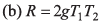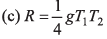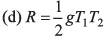Ans: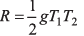Sol: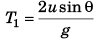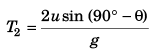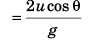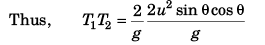or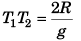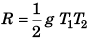Option (d) is correct.

Ques 9: A grass hopper can jump maximum distance 1.6 m. It spends negligible time on ground. How far can it go in 10√2 s.
(a) 45 m
(b) 30 m
(c) 20 m
(d) 40 m
Ans: 40 m
Sol: Rmax = 1.6 m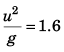⇒ u = 4 m/s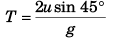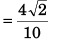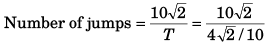= 25
∴ Grass hopper would go = 25 x 1.6 m i.e., 40 m.
Option (d) is correct.

Ques 10: Average velocity of a particle in projectile motion between its starting point and the highest point of its trajectory is (projection speed = u, angle of projection from horizontal = θ)
(a) u cos θ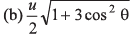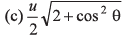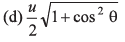Ans: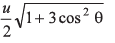Sol: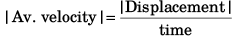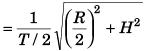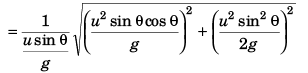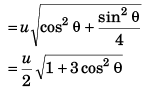Option (b) is correct.

Ques 11: A train is moving on a track at 30 ms-1. A ball is thrown from it perpendicular to the direction of motion at 30 ms-1 at 45° from horizontal. Find the distance of ball from the point of projection on train to the point where it strikes the ground.
(a) 90 m
(b) 90 √3 m
(c) 60 m
(d) 60 √3 m
Ans: 90 m
Sol: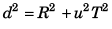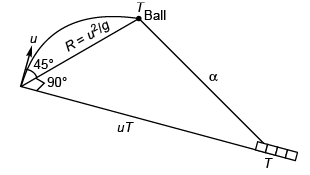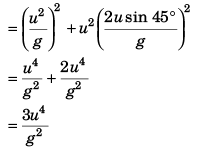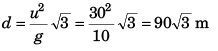Option (b) is correct.

Ques 12: A body is projected at time t = 0 from a certain point on a planet’s surface with a certain velocity at a certain angle with the planet’s surface (assumed horizontal). The horizontal and vertical displacements x and y (in metre) respectively vary with time t in second as, x = (10√3) 7 and y = 10t - t2. The m axim um height attained by the body is
(a) 200 m
(b) 100 m
(c) 50 m
(d) 25 m
Ans: 25 m
Sol: At maximum height
dy/dt = 0
i.e.,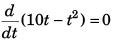or   10 - 2t = 0
or t = 5 s
∴ Maximum height attained = 10 (5) - 52
= 25 m
Option (d) is correct.

Ques 13: A particle is fired horizontally from an inclined plane of inclination 30° with horizontal with speed 50 ms-1. If g = 10 ms-2, the range measured along the incline is
(a) 500 m
(b)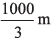(c)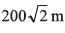(d)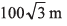Ans: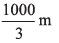Sol: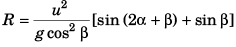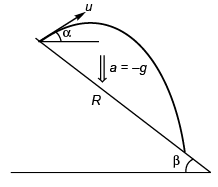As α = 0 (according to question)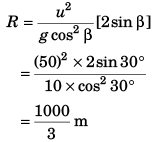Option (b) is correct.

Ques 14: Two stones are projected with the same speed but making different angles with the horizontal. Their horizontal ranges are equal. The angle of projection of one is π/3 and the maximum height reached by it is 102 m. Then the maximum height reached by the other in metres is
(a) 336
(b) 224
(c) 56
(d) 34
Ans: 34
Sol: First particle:
Hmax = 102 m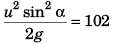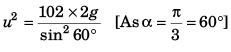Second particle:
Range of the second particle will be equal to that of particle
if,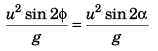sin 2φ = sin 3α
2φ = π - 2α
or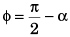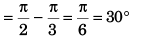∴ Maximum height attained by second particle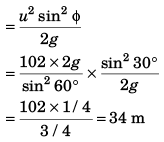Option (d) is correct.

Ques 15: A ball is projected upwards from the top of a tower with a velocity 50 ms-1 making an angle 30° with the horizontal. The height of tower is 70 m. After how many seconds from the instant of throwing, will the ball reach the ground, (g = 10 ms-2
(a) 2 s
(b) 5 s
(c) 7 s
(d) 9 s
Ans: 7 s
Sol: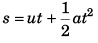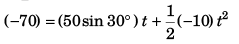or 5t2 - 25t - 70 = 0
or t2 - 5t - 14 = 0
(t - 7) (t + 2) = 0
t = 7 s
(- 2 s not possible)
Option (c) is correct.

Ques 16: A fixed mortar fires a bomb at an angle of 53° above the horizontal with a muzzle velocity of 80 ms-1. A tank is advancing directly towards the mortar on level ground at a constant speed of 5m/s. The initial separation (at the instant mortar is fired) between the mortar and tank, so that the tank would be hit is [Take g = 10 ms-2
(a) 662.4 m
(b) 526.3 m
(c) 486.6 m
(d) None of these
Sol: Initial separation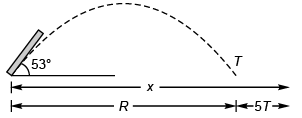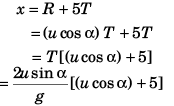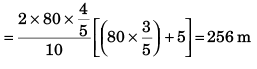Option (d) is correct.

Offer running on EduRev: Apply code STAYHOME200 to get INR 200 off on our premium plan EduRev Infinity!

210 docs

,

,

,

,

,

,

,

,

,

,

,

,

,

,

,

,

,

,

,

,

,

,

,

,

;